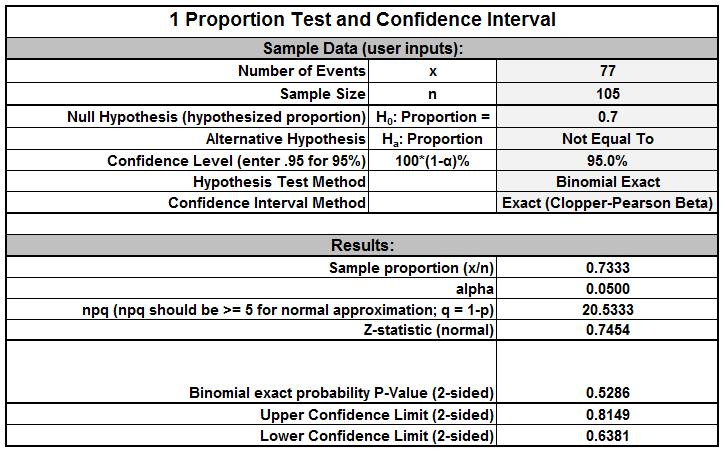# One Sample Proportion Test with SigmaXL

### What is the One Sample Proportion Test with SigmaXL?

One sample proportion test with SigmaXL is a hypothesis test to compare the proportion of one certain outcome (e.g. the number of successes per the number of trials, or the number of defects per the total number of opportunities) occurring in a population following the binomial distribution with a specified proportion.

• Null Hypothesis (H0): p = p0
• Alternative Hypothesis (Ha): pp0

### One Sample Proportion Test Assumptions

• The sample data drawn from the population of interest are unbiased and representative.
• There are only two possible outcomes in each trial: success/failure, yes/no, and defective/non-defective etc.
• The underlying distribution of the population is binomial distribution.
• When np ≥ 5 and np(1 – p) ≥ 5, the binomial distribution can be approximated by the normal distribution.

### How the One Sample Proportion Test Works

When np ≥ 5 and np(1 – p) ≥ 5, we use the normal distribution to approximate the underlying binomial distribution of the population.

When |Zcalc| (absolute value of the calculated test statistic) is smaller than Zcrit (critical value), we fail to reject the null hypothesis and claim that there is no statistically significant difference between the population proportion and the hypothesized proportion.

### Run a One Sample Proportion Test with SigmaXL

Case study: We are interested in comparing the exam pass rate of a high school this month against a specified rate (70%) using a nonparametric (i.e. distribution-free) hypothesis test: one sample proportion test.
Data File: “One Sample Proportion” tab in “Sample Data.xlsx”

• Null Hypothesis (H0): p = 70%
• Alternative Hypothesis (Ha): p ≠ 70%

Steps to run a one sample proportion test in SigmaXL:

1. Click SigmaXL -> Statistical Tools -> Basic Statistical Templates -> 1 Proportion Test and Confidence Interval
2. A new tab named “1 Proportion Test CI” appears – use this template to enter the following:
3. Enter “77” in the yellow box of “Number of Events in category of interest x”
4. Enter ‘105” in the yellow box of “Sample Size”
5. Enter 0.70 as “hypothesized proportion” test against 70%
6. Keep 95% as the default confidence levelModel summary: The p-value of the one sample proportion test is 0.5268, greater than the alpha level (0.05), and we fail to reject the null hypothesis. We conclude that the exam pass rate of the high school this month is not statistically different from 70%.